## Example to show how numerical ODE solutions can be used to find integrals

In a previous post, I enumerated how we can use numerical ODE techniques like Euler and Runge-Kutta methods to find approximate value of definite integrals. Here is an example. Be sure to do the exercises at the end of the post to appreciate the procedure.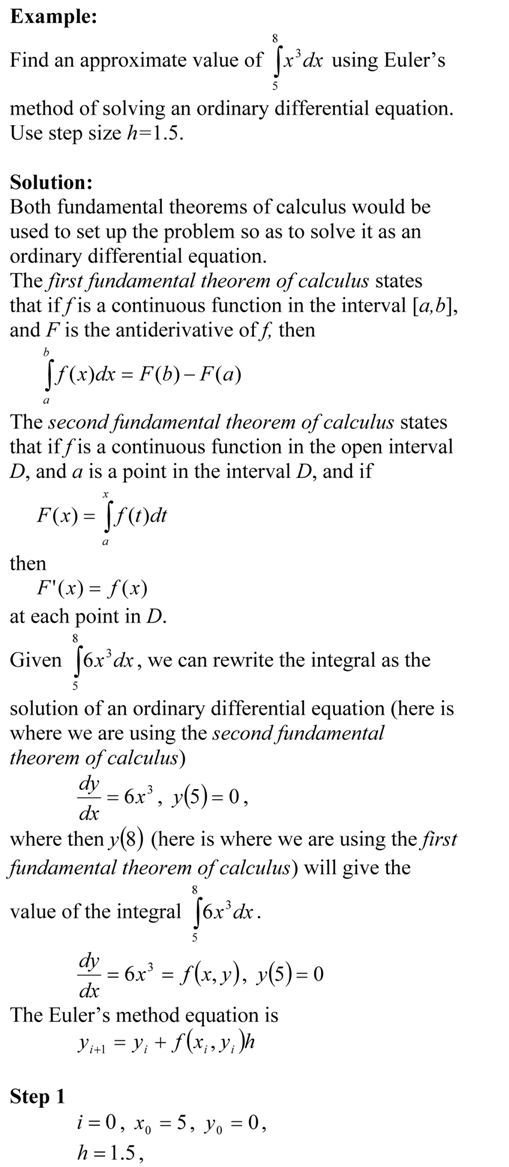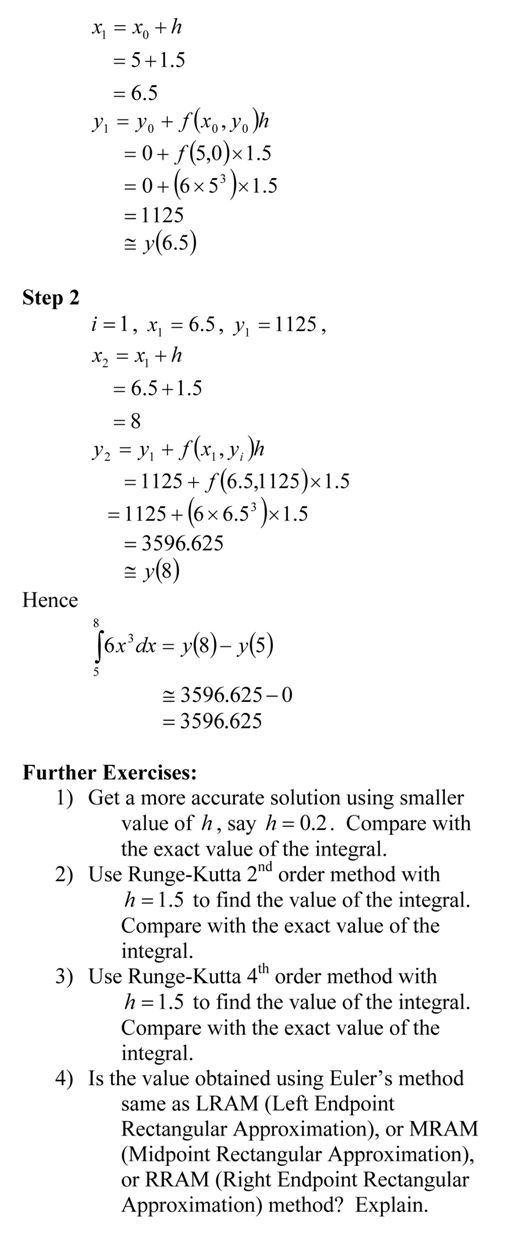_____________________________________________________

This post is brought to you by Holistic Numerical Methods: Numerical Methods for the STEM undergraduate at http://nm.mathforcollege.com

## Comparing Runge-Kutta 2nd order methods

Many a times, students ask me

Which of the Runge-Kutta 2nd order methods gives the most accurate answer to solving a first order ODE?

dy/dx=f(x,y), y(0)=y0

There is no direct answer, although Ralston’s method gives a minimum bound for the truncation error (Ralston, A., Runge-Kutta Methods with Minimum Error Bounds, Match. Compu., Vol 16, page 431, 1962).

They also ask me if using the first three terms of the Taylor series would give a more accurate answer if we calculate $f^{\prime}(x,y)$ symbolically.

The equations for the four methods are given below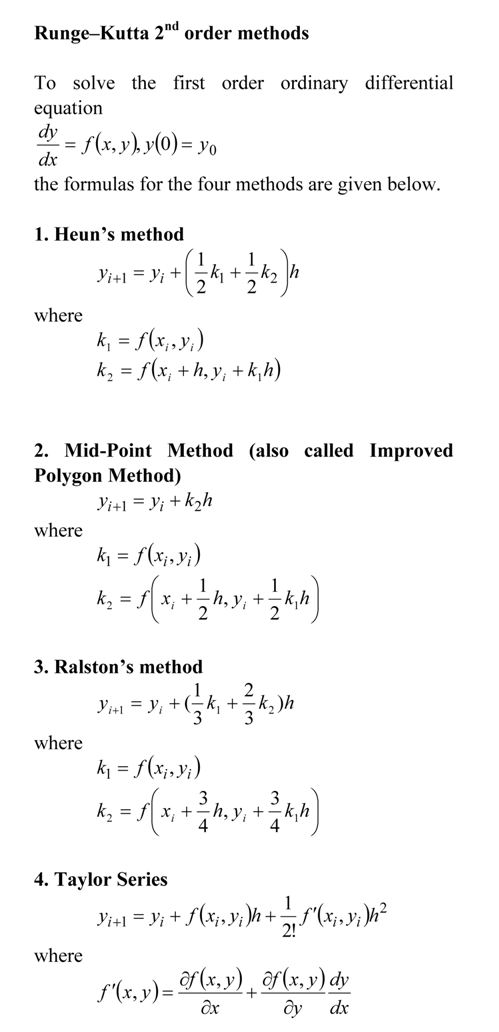Here is the comparison graph for

dy/dx=sin(5*x)-0.4*y, y(0)=5

with

step size of h=1.1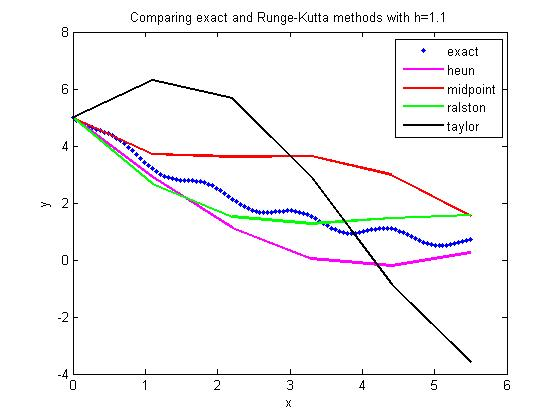and

step size of h=0.55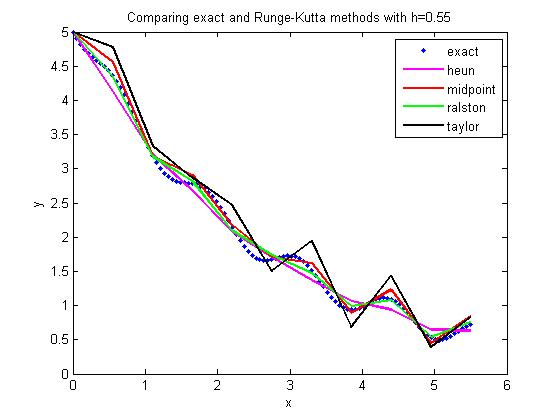This post is brought to you by Holistic Numerical Methods: Numerical Methods for the STEM undergraduate at http://nm.mathforcollege.com

## Can I use numerical solution of ODE techniques to do numerical integration?

Yes.

If you are finding the value of the $latex y=\int_{a}^{b} f(x) dx$, then we can solve the integral as an ordinary differential equation as

dy/dx=f(x), y(a)=0

We can now use any of the numerical techniques such as Euler’s methods and Runge-Kutta methods to find the value of y(b) which would be the approximate value of the integral. Use exact techniques of solving linear ODEs with fixed coefficients such as Laplace transforms, and you can have the exact value of the integral.

_____________________________________________________

This post is brought to you by Holistic Numerical Methods: Numerical Methods for the STEM undergraduate at http://nm.mathforcollege.com

## Time of death – a classic ODE problem

One of the classical applied problems in ordinary differential equations is that of finding the time of death of a homicide victim.

The estimation of time of death is generally based on the temperature of the body at two times – 1) when the victim is found and 2) then a few hours later. Assuming the ambient temperature stays the same and the body is treated as a lumped system, one can use a simple linear ODE to solve the problem.

It is somewhat an inverse problem as we are trying to find the value of the independent variable – the time of death. Here is a solved problem (a pdf version also available).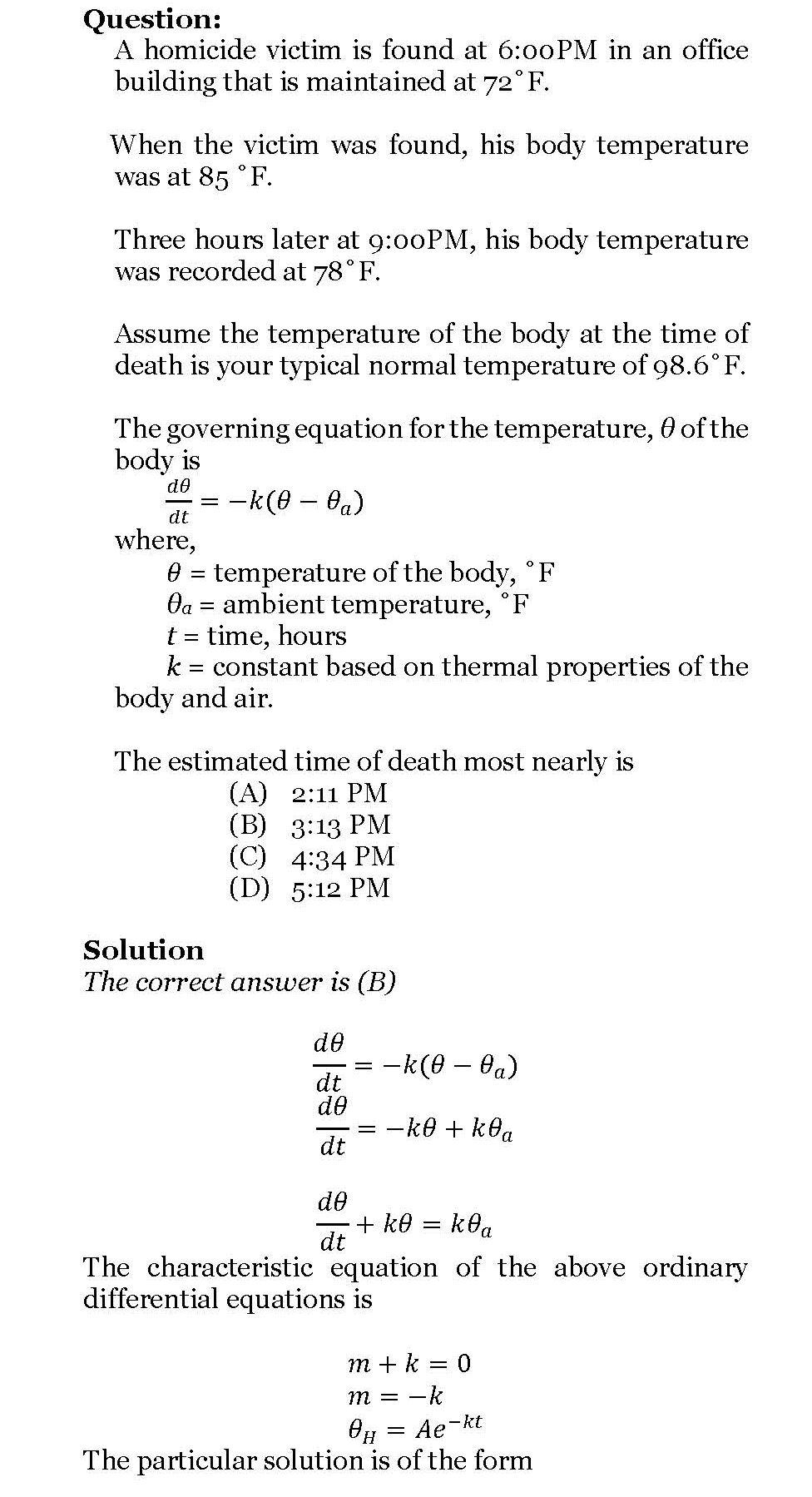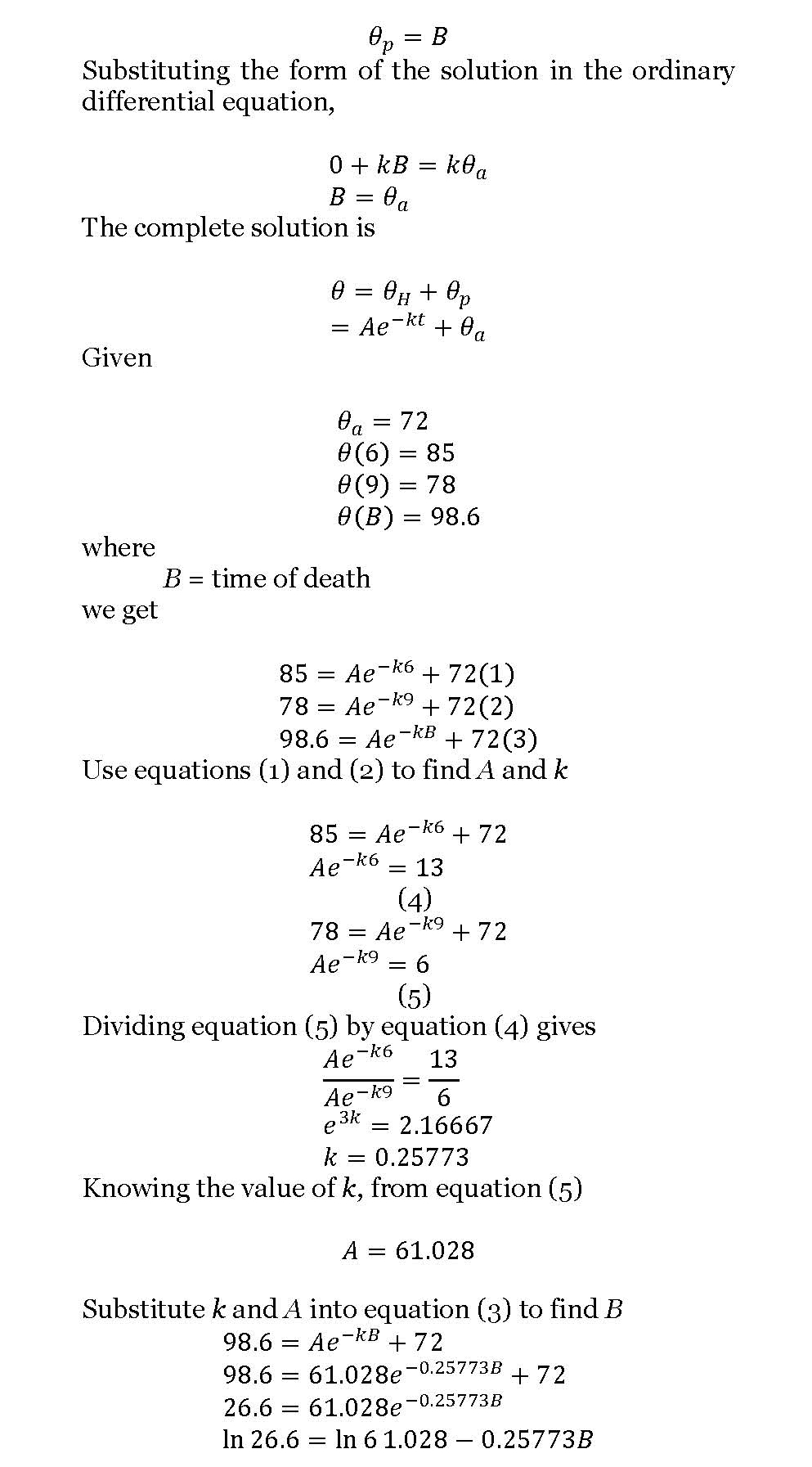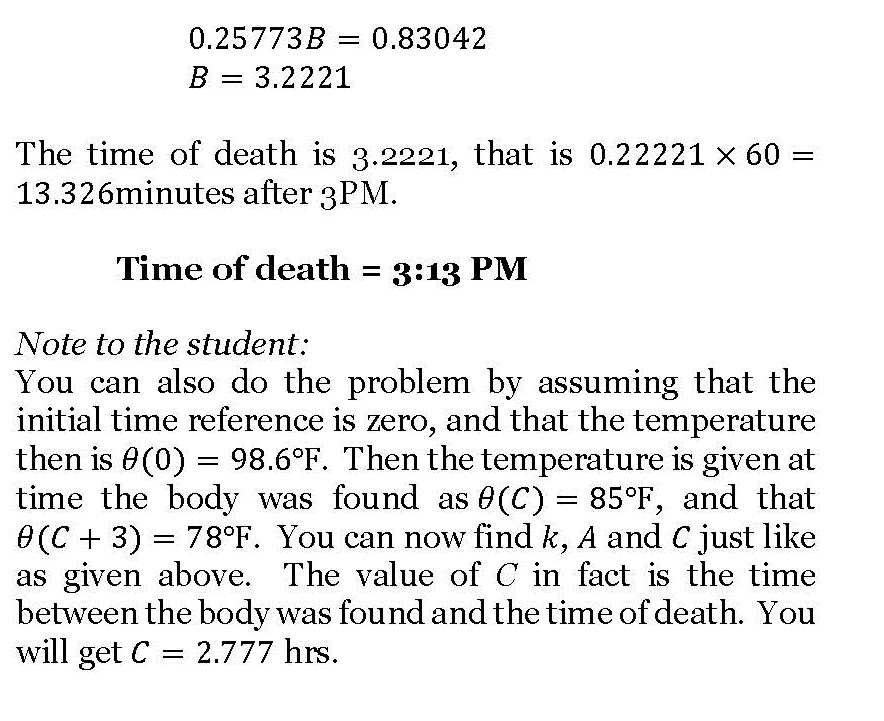_____________________________________________________

This post is brought to you by Holistic Numerical Methods: Numerical Methods for the STEM undergraduate at http://nm.mathforcollege.com

## Is it just a coincidence – true error in multiple segment Trapezoidal rule gets approximately quartered as the number of segments is doubled?

Look at the table below. This is a table that shows the approximate value of the integral

$latex \int_{8}^{30} 2000 ln \frac{140000}{140000-2100t}-9.8t dt$

as a function of the number of segments used in the Trapezoidal rule and the corresponding true error.

 n Value Et 1 11868 -807 2 11266 -205 3 11153 -91.4 4 11113 -51.5 5 11094 -33.0 6 11084 -22.9 7 11078 -16.8 8 11074 -12.9

The true error for n=1 is -807 and for n=2 is -205. As you can see the quarter of -807 is approximately -201.75 and close to the true error for n=2. Is this a coincidence?

Look at the true error for n=2 which is -205 and for n=4 is -51.5. As you can see the quarter of -205 is approximately -51.75 and close to the value of the true error for n=4. Is this a coincidence?

No. This is because the true error in a single segment trapezoidal rule is

$latex \frac{(b-a)^3}{12} f^{\prime\prime} (c)$

where c is some point not known but in the domain [a,b] of $latex \int_{a}^{b} f(x) dx$. It can be then shown (see page 14 of this pdf file for full proof) that for the multiple segment trapezoidal rule, the true error is

$latex \frac{(b-a)^3}{12n^2} f^{\prime\prime}$

where the $latex f^{\prime\prime}$ is an average value of the second derivative of the function f(x) calculated at some point within each of the n segments. Since a and b are constant, and $latex f^{\prime\prime}$ becomes almost a constant as n increases, the true error is approximately inversely proportional to the square of the number of segments.

Note to the reader: Develop a similar table as given above for an integral of your choice and see it for yourself if the true error gets approximately quartered as the number of segments is doubled.

_____________________________________________________

This post is brought to you by Holistic Numerical Methods: Numerical Methods for the STEM undergraduate at http://nm.mathforcollege.com

## Can I use Trapezoidal rule to calculate an improper integral?

For example, $latex \int_{a}^{infinity} e^{-x} dx$ is an improper integral which can be calculated exactly as $latex e^{-a}$.

Can we solve this integral by multiple segment Trapezoidal rule when we already know that the upper limit is infinity?

Yes, we can solve it in spite of the upper limit being infinity. We first need to make a change of variables such as $latex t=\frac{1}{x}$, then $latex dx=-\frac{1}{t^2}dt$ giving $latex \int_{1/a}^{0} e^{-1/t} (-1/t^2) dt$

But even for this integral we have issues as the integrand gives division by zero problems at t=0 (although the limit itself is zero at t=0).

The MATLAB program that can be downloaded at http://nm.mathforcollege.com/blog/trapezoidal_improper.m (better to download it as the single quotes do not translate well with matlab editor) shows that we get accurate results for transformed integral with 256 segments.
% Simulation : Can I use Trapezoidal rule for an improper integral?
% Language : Matlab 2007a
% Authors : Autar Kaw, http://nm.mathforcollege.com
% mfile available at
% http://nm.mathforcollege.com/blog/trapezoidal_improper.m
% Last Revised : July 15, 2008
% Abstract: This program shows use of multiple segment Trapezoidal
% rule to integrate exp(-x) from x=a to infinity, where a>0.
clc
clear all
disp(‘This program shows the convergence of getting the value of ‘)
disp(‘an improper integral using multiple segment Trapezoidal rule’)
disp(‘Author: Autar K Kaw. autarkaw.wordpress.com’)

%INPUTS. If you want to experiment, these are the only variables
% you should and can change.
% a = Lower limit of integration
a=1/2;

fprintf(‘\nFinding the integral of exp(-x) from a to infinity where a=%g’,a)

% SIMULATION
syms x
% integrand exp(-x)
f=exp(-x);
valexact=double(int(f,x,a,inf));
fprintf(‘\n\nExact value of integral = %f’,valexact)
disp( ‘ ‘)

% Transformed integrand by change of variables
ft=inline(‘exp(-1/t)*(-1/t^2)’);
% Limits of integration
at=1/a;
b=0;

%finding value of the integral using 16, 32, 64, 128 and 256 segments
for k=4:1:8
n=2^k;
h=(b-at)/n;
sum=0;
for i=1:1:n-1
sum=sum+ft(at+i*h);
end
% See how f(b) is not added as f(b)=infinity. But
% using a different value of the function at one point or
% finite number of points does not change the value of the
% integral. We are substituting f(b)=0
sum=2*sum+ft(at)+0;
sum=(b-at)/(2*n)*sum;
fprintf(‘\nApproximate value of integral =%f with %g segments’,sum,n)
end
disp(‘ ‘)
This program shows the convergence of getting the value of
an improper integral using multiple segment Trapezoidal rule
Author: Autar K Kaw. autarkaw.wordpress.com

Finding the integral of exp(-x) from a to infinity where a=0.5

Exact value of integral = 0.606531

Approximate value of integral =0.605971 with 16 segments
Approximate value of integral =0.606496 with 32 segments
Approximate value of integral =0.606521 with 64 segments
Approximate value of integral =0.606528 with 128 segments
Approximate value of integral =0.606530 with 256 segments

Published with MATLAB® 7.3

Now someone may rightly point out that it works well because the limit of the integrand is zero at the lower limit of integration for the above mentioned integral.

So go ahead, modify the program for doing this improper integral $latex \int_{0}^{4} \frac{1}{\sqrt {x}} dx$ and see how well it works. In this case the integrand is singular at $latex x=0$ and one would have to assume the function to be a number other than infinity at $latex x=0$ for applying the multiple segment Trapezoidal rule.

How many segments do you need to get an accurate answer for this integral?

_____________________________________________________

This post is brought to you by Holistic Numerical Methods: Numerical Methods for the STEM undergraduate at http://nm.mathforcollege.com

## A metric for measuring wildness of a college football season

A Metric to Quantify the Topsy-Turvyness (Wildness) of a College Football Season

The 2008 college football season is almost here, and news media, sports commentators, and bloggers will hope for something to hype about. Luckily for them, the 2007 season did give them something to talk about; you would be hard pressed to recall a more topsy-turvy season. Ranked teams regularly lost to low-ranked and unranked teams.

In just Week#1 of the 2007 season, Associated Press (AP) No. 5 team University of Michigan lost to an unranked Division II team – Appalachian State. The Associated Press wasted no time in booting out Michigan out of the Top AP 25. Two weeks later, No. 11 UCLA lost to unranked Utah by a wide margin of 44-6. UCLA also met the same fate as Michigan; UCLA was dropped from the AP Top 25.

The topsy-turvyness continued in the season, especially for No. 2 ranked teams. The University of South Florida, where I work, was ranked No. 2 when they lost to unranked Rutgers 30-27 in Week#8. This was the same week when three other teams (South Carolina, Kentucky, and California) ranked in the Top 10 of the AP poll also lost their games.

To top off the season, for the first time in history of the Bowl Championship Series (BCS), the title bowl game had a team (Louisiana State University (LSU)) with two regular season losses, and LSU ended up winning the national championship.

Although many ranted and raved about the anecdotal evidence of a topsy-turvy season, is it possible that the media and fans over exaggerated the topsy-turvyness of the 2007 college football season. Were there other seasons that were more topsy-turvy than 2007?

To answer this question scientifically, this article proposes an algorithm to quantify the topsy-turvyness of the college football season. The author does not know of any previous literature that has attempted to develop a metric that quantifies the topsy-turvyness of any sport, which is ranked regularly in the season.

The TT factor

The Topsy-Turvy Factor (TT factor) is a metric that quantifies the topsy-turvyness of a college football season. Two different TT factors are calculated: one for each of week of the season, referred to as the Week TT factor, and one for the cumulative topsy-turvyness at the end of each week of the season, referred to as the Season TT factor.

The method to find the Week TT Factor is based on comparing the AP Top 25 poll rankings of schools from the current week to that of the previous week. The difference in the rankings of each school in the AP Top 25 from the current week to the previous week is squared. How do we account for teams that fall out of the rankings? A team that gets unranked from the previous week is assumed as having become the No. 26 team in the current week. All the squares of the differences in the rankings are then added together and normalized on a scale of 0-100.

The other TT factor, the Season TT factor is also calculated for the end of each week to gage how topsy-turvy the season has been so far. The Season TT factor is calculated using weighted averages of the Week TT factors. As the season progresses, the Week TT factors are given more weight in the calculation of the Season TT factor because toward the end of the season, an upset of a ranked team is more topsy-turvy than an upset in the beginning of the season when the strength of a ranked team is less established.

Season End of Season TT factor
2007 ……………..60
2006 ……………..46
2005 ……………..48
2004 ……………..40
2003 ……………. 55
2002 ……………. 48

Table 1: End of Season TT factors for 2002-2007 Seasons

Table 1 shows the end of Season TT factor of the last six football seasons. It is clear that 2007 was the most topsy-turvy season in recent history, with the 2003 season not too far behind. In contrast, the 2004 season was the least topsy-turvy.

Read the complete paper including formulas and detailed analysis at

http://www.eng.usf.edu/~kaw/TT_factor_paper_media.pdf

Can you write a program in MATLAB or any other language to find the Week TT factors and the Season TT factors?

_____________________________________________________

This post is brought to you by Holistic Numerical Methods: Numerical Methods for the STEM undergraduate at http://nm.mathforcollege.com

## Experiment for spline interpolation and integration

Background:

The motivation behind the experiment is to understand spline interpolation and numerical integration by finding the volume of water that can be held by a champagne glass.

What does the student do in the lab:

The student chooses one of the odd-shaped champagne glasses (Figure 1). The student measures the outer radius of the champagne glass at different known locations along the height. The student measures the thickness of the glass, so that he/she will be able to find the inner radius of the champagne glass at the locations he/she measured the outer radius. The student pours water to the brim in the champagne glass and checks how much volume the champagne glass holds.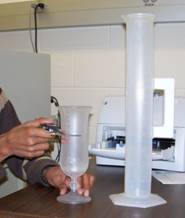Exercises assigned to the students:
Use MATLAB to solve problems. Use comments, display commands and fprintf statements, sensible variable names and units to explain your work. Staple all the work in the following sequence. Use USCS system of units throughout.

1. Attach the data sheet on which you collected the data in class.
2. Find the spline interpolant that curve fits the radius vs height data.
3. Show the individual points and the spline interpolant of radius vs height on a single plot.
4. Find how much volume of water the champagne glass would hold.
5. Compare the above result from problem#4 to the actual volume.
6. In 100-200 words, type out your conclusions using a word processor. Any formulas should be shown using an equation editor. Any sketches need to be drawn using a drawing software such as Word Drawing. Any plots can be imported from MATLAB.

What materials do you need; where do I buy it; how much do the materials costs?

1. Champagne Glasses: These glasses, called the Hurricane Plastic Glasses, are available at www.poolsidepineapple.com, part nos. HUR-105, HUR-106, YAR-114. We used glasses made of plastic to avoid breakage. http:/www.poolsidepineapple.com/cart_pages/shopping%20page%20tropical.htm. You can try other places to buy the champagne glasses. About $40 or so for about six pieces including S&H. Better yet, go to a cruise and get souvenir glasses. Whenever you do the experiment, you will remember the good times. 2. Graduated Cylinder: The graduated cylinder is available at http://scientificsonline.com/, part number 3036286. The cost of the cylinder is$20+S&H.
3. Vernier Caliper: The caliper is available at http://mcmaster.com part number 20265A49. The cost of the vernier caliper is $60+S&H. 4. Scale: Need to buy a thin scale for this. Any art-supplies store for$2 or so.

_____________________________________________________

This post is brought to you by Holistic Numerical Methods: Numerical Methods for the STEM undergraduate at http://nm.mathforcollege.com

## Abuses of regression

There are three common abuses of regression analysis.

1. Extrapolation
2. Generalization
3. Causation.

Extrapolation

If you were dealing in the stock market or even interested in it, we remember the stock market crash of March 2000. During 1997-1999, many investors thought they would double their money every year, started buying fancy cars and houses on credit, and living the high life. Little did they know that the whole market was hyped on speculation and little economic sense? Enron and MCI financial fiascos were soon to follow.

Let us look if we could have safely extrapolated NASDAQ index from past years. Below is the table of NASDAQ index, S as a function of end of year number, t (Year 1 is the end of year 1994, and Year 6 is the end of year 1999).

Table 1 NASDAQ index as a function of year number.

 Year Number (t) NASDAQ Index (S) 1 (1994) 752 2 (1995) 1052 3 (1996) 1291 4 (1997) 1570 5 (1998) 2193 6 (1999) 4069

A relationship S = a0+a1t+a2t2 between the NASDAQ index, S and the year number, t is developed using least square regression and is found to be

S=168.14t2 – 597.35t + 1361.8

The data is given for Years 1 thru 6 and it is desired to calculate the value for t>=6. This is extrapolation outside the model data. The error inherent in this model is shown in Table 2. Look at the Year 7 and 8 that was not included in the regression data – the error between the predicted and actual values is 119% and 277%, respectively.

Table 2 NASDAQ index as a function of year number.

 Year Number (t) NASDAQ Index (S) Predicted Index Absolute Relative True Error (%) 1 (1994) 752 933 24 2 (1995) 1052 840 20 3 (1996) 1291 1082 16 4 (1997) 1570 1663 6 5 (1998) 2193 2578 18 6 (1999) 4069 3831 6 7 (2000) 2471 5419 119 8 (2001) 1951 7344 277

This illustration is not exaggerated and it is important that a careful use of any given model equations is always called for. At all times, it is imperative to infer the domain of independent variables for which a given equation is valid.

Generalization

Generalization could arise when unsupported or overexaggerated claims are made. It is not often possible to measure all predictor variables relevant in a study. For example, a study carried out about the behavior of men might have inadvertently restricted the survey to Caucasian men. Shall we then generalize the result as the attributes of all men irrespective of race? Such use of regression equation is an abuse since the limitations imposed by the data restrict the use of the prediction equations to Caucasian men.

Misidentification

Finally, misidentification of causation is a classic abuse of regression analysis equations. Regression analysis can only aid in the confirmation or refutation of a causal model ‑ the model must however have a theoretical basis. In a chemical reacting system in which two species react to form a product, the amount of product formed or amount of reacting species vary with time. Although a regression equation of species concentration and time can be obtained, one cannot attribute time as the causal agent for the varying species concentration. Regression analysis cannot prove causality; rather they can only substantiate or contradict causal assumptions. Anything outside this is an abuse of the use of regression analysis method.

This post used textbook notes written by the author and Egwu Kalu, Professor of Chemical and Biomedical Engineering, FAMU, Tallahassee, FL.

____________________________________________________

This post is brought to you by Holistic Numerical Methods: Numerical Methods for the STEM undergraduate at http://nm.mathforcollege.com

## How do you know that the least squares regression line is unique and corresponds to a minimum

We already know that using the criterion of either

1. minimizing sum of residuals OR
2. minimizing sum of the absolute value of residuals

is BAD as either of the criteria do not give a unique line. Visit these notes for an example where these criteria are shown to be inadequate.

So we use minimizing the sum of the squares of the residuals as the criterion. How can we show that this criterion gives a unique line?

The proof is given below as image files because the proof is equation intensive. I made a better resolution pdf file also.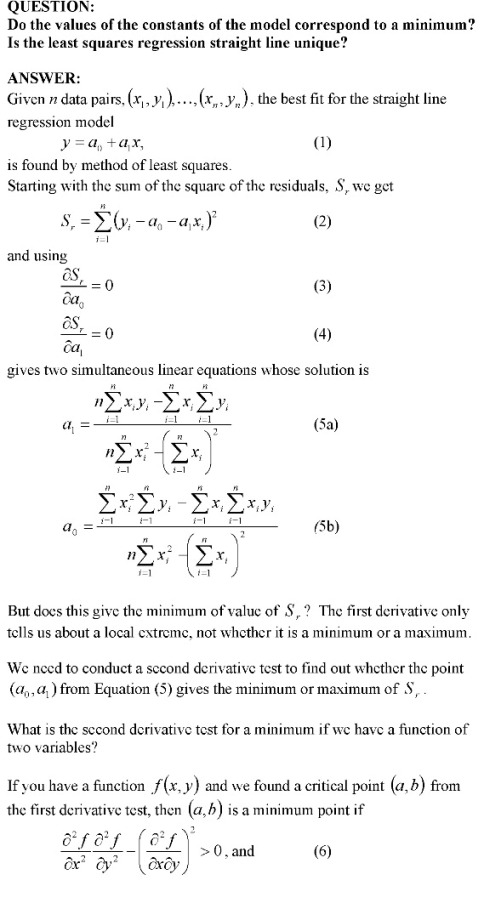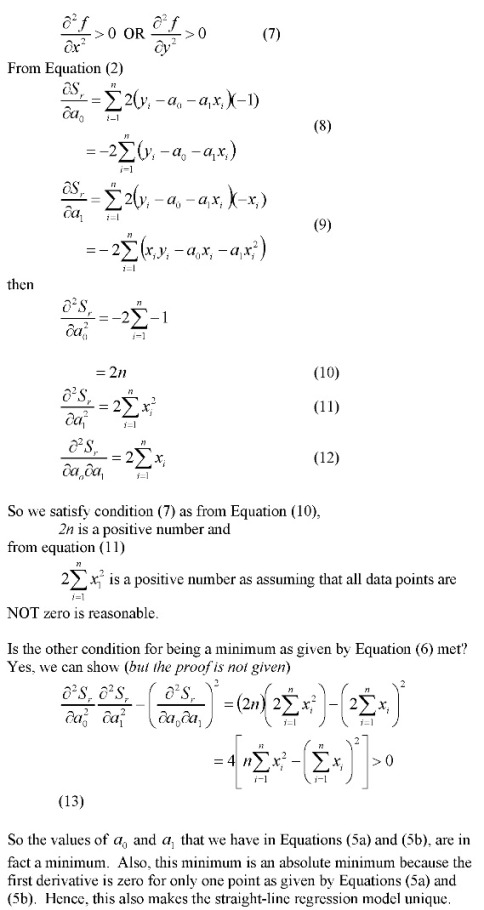_____________________________________________________

This post is brought to you by Holistic Numerical Methods: Numerical Methods for the STEM undergraduate at http://nm.mathforcollege.com